Next: F.6 Correction of Spatial Up: Calibration Files for Standard Previous: F.4 Temporal Gain Correction

# F.5 Electronic Position Correction (EPC)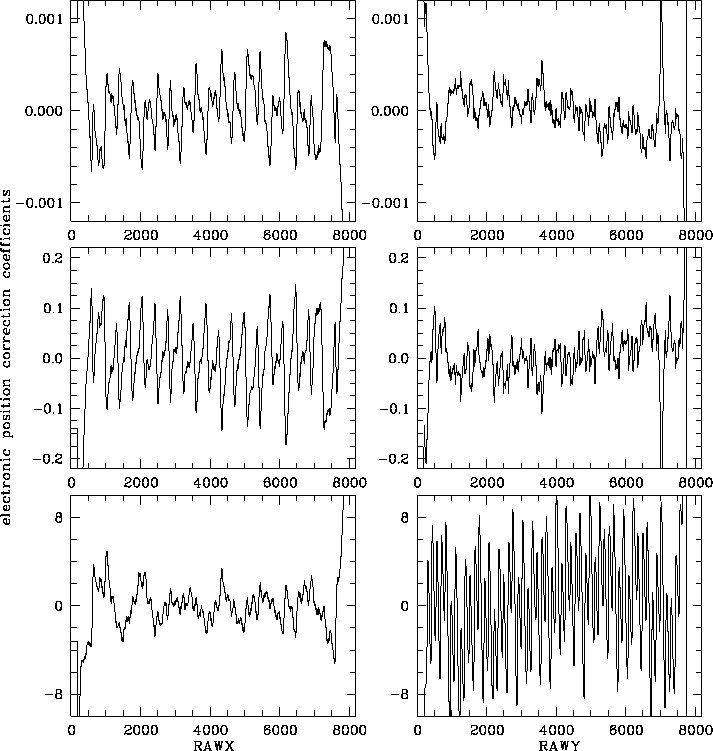Figure F.6: Electronic position correction tables for PSPC-C: left from top to bottom,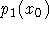, and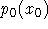, according to Eq.(F.8); right from top to bottom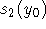,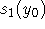, and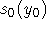, according to Eq.(F.9). The recorded raw detector coordinates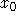and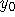correspond to RAWX and RAWY in the figure.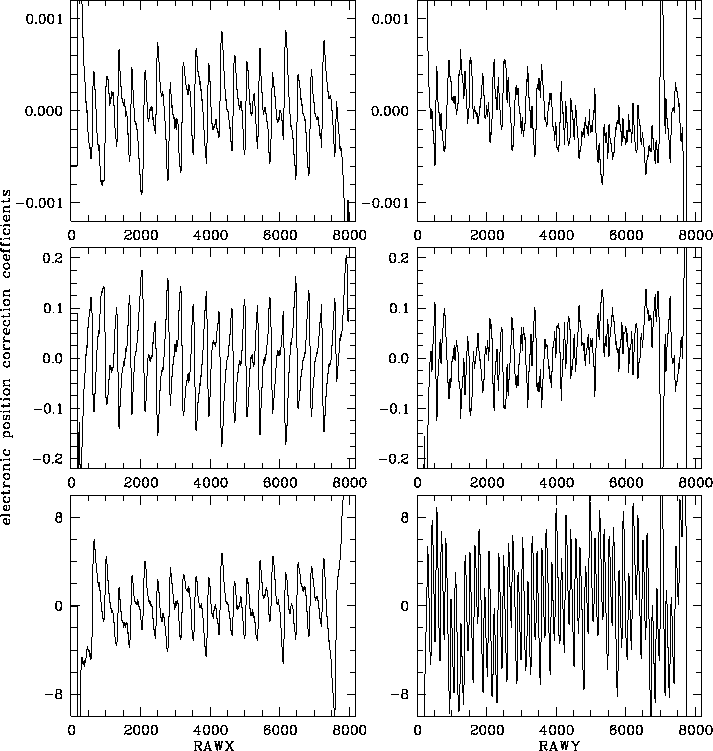Figure F.7: Electronic position correction tables for PSPC-B: left from top to bottom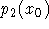,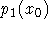, and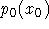, according to Eq.(F.8); right from top to bottom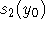,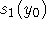, and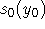, according to Eq.(F.9). The recorded raw detector coordinatesandcorrespond to RAWX and RAWY in the figure.

The PSPC wires cause small-scale spatial non-linearities. This effect depends on the position as well as on the energy of the photon. A correction vector is computed using a parabola with spatially-dependent coefficients and the amplitude as variable according to Eq. (F.8) and (F.9).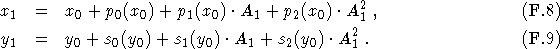Here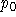,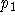, and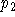are the correction coefficients parallel to the anode wire direction while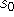,, and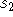denote coefficients perpendicular (``senkrecht'') to the anode wires. The correction coefficients are stored in table with 4000 elements and the electronic position correction is actually done by interpolation between two table elements (cf. Eq. (F.11)).

Figure F.6 and F.7 shows this correction for PSPC-C and PSPC-B, respectively.

There is an inconsistency in the documentation about the amplitude to be used in the correction: [Hasinger and Snowden1990] as well as Chap. 3 and [Turner et al.1995] state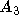, whereas Chap. 8 (SASS) uses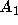. In fact, in the creation of the correction tables no corrections had been applied, i.e.,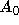should be used; however, the differential effect betweenandis minor [Briel1995].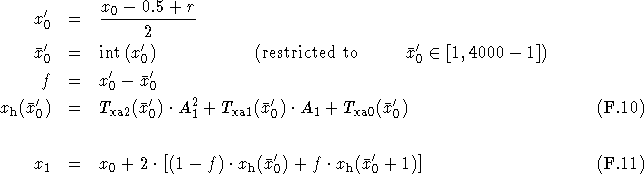Parameters:] raw detector x coordinate (integer number in [1,8192])pulse height of event, corrected for ADC non-linearity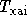tables for electronic position correction; dimension 4000 (Figs. F.6 and F.7, interpolated)
r
random number (real number in [0.0,1.0])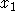detector x coordinate (electronic position correction applied)

The electronic position correction in y is done similiar to the correction in x; simply replace x by y in the above formulae.

Remarks:as input to DCORE.

SASS source code: package PSPC, bubble CT, subroutine DCORE

SASS files:x direction (PSPC-C) (Fig. F.6)
p\$manpar:senk_c.dat
correction tables for y direction (PSPC-C) (Fig. F.6)
p\$manpar:para_b.dat
correction tables for x direction (PSPC-B) (Fig. F.7)
p\$manpar:senk_b.dat
correction tables for y direction (PSPC-B) (Fig. F.7)Next: F.6 Correction of Spatial Up: Calibration Files for Standard Previous: F.4 Temporal Gain Correction

If you have problems/suggestions please send mail to rosat_svc@mpe-garching.mpg.de